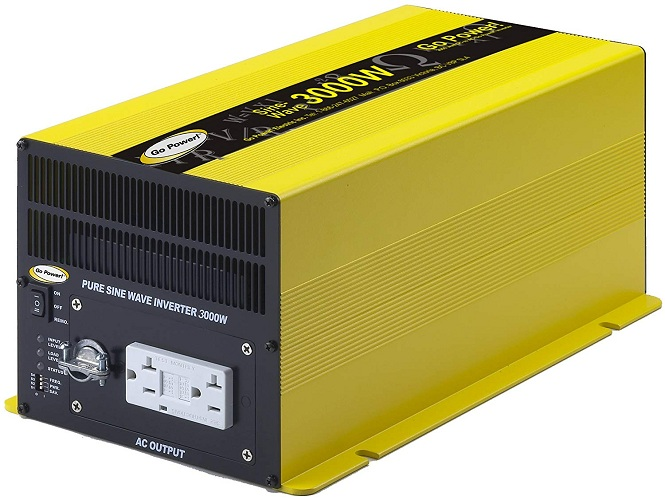# Inverter load capacity: What you need to know

Introduction

An inverter is an electrical device that converts DC (direct current) power into AC (alternating current) power, allowing you to use AC-powered devices wherever you are, whether you’re camping or experiencing a power outage at home. However, not all inverters are created equal, and understanding their load capacity is crucial in ensuring that you choose the right inverter for your needs.In this article, we’ll dive into the concept of inverter load capacity, exploring what it means, why it’s important, the factors that affect it, how to calculate it, and tips for choosing the right inverter.

Inverter load capacity refers to the maximum amount of power an inverter can supply to your electrical load. It is measured in watts and is typically expressed as two different values: continuous load capacity and surge load capacity.

1. Continuous load capacity: This refers to the maximum amount of power the inverter can supply to the load over a prolonged period. It is important to choose an inverter that can handle the continuous power requirements of your load.
2. Surge load capacity: This refers to the maximum amount of power the inverter can supply to the load for a brief moment, usually for starting electric motors or other devices that require a sudden surge of power. Surge load capacity is typically higher than the continuous load capacity and is an important factor to consider when choosing an inverter for devices with high starting currents.

## III. Why is inverter load capacity important?

1. Consequences of overloading the inverter: When an inverter is overloaded, it may shut down or even become damaged. Overloading the inverter can also cause a decrease in the output voltage, which can damage sensitive devices such as laptops or smartphones.

Several factors can affect an inverter’s load capacity, including:1. Ambient temperature: High temperatures can reduce the inverter’s capacity due to increased internal resistance, leading to lower efficiency and potentially damaging the inverter.
2. Battery voltage: Inverter load capacity is also affected by the battery voltage. As the battery voltage drops, the inverter’s output voltage will decrease, potentially causing damage to the device.
3. Type of load: Different loads have different power requirements and may require different types of inverters. For example, a resistive load, such as a heater, will have a lower starting current and require less surge capacity than an inductive load, such as a motor.
4. Inverter efficiency: The efficiency of the inverter can also affect its load capacity. A higher efficiency inverter can supply more power to the load while drawing less power from the battery.

## Calculating the inverter load capacity

1. How to calculate continuous load capacity: To calculate the continuous load capacity, you need to add up the wattage of all the devices you plan to power with the inverter. For example, if you plan to power a refrigerator (200 watts), a laptop (60 watts), and a light bulb (40 watts), your total load would be 300 watts. You should choose an inverter with a continuous load capacity that is greater than 300 watts.
2. How tocalculate surge load capacity: Calculating surge load capacity requires an understanding of the type of load you’re powering. Typically, you’ll need to multiply the continuous load capacity by a factor of two to three for devices that require a sudden surge of power to start, such as motors or compressors.

For example, if your continuous load is 300 watts, you may need an inverter with a surge load capacity of 600 to 900 watts for devices that require a surge of power to start.

## Tips for choosing the right inverter

Choosing the right inverter can be daunting, but these tips can help you make an informed decision:1. Determine the wattage of the load: As discussed earlier, you need to know the wattage of the devices you plan to power to calculate the inverter’s load capacity.
2. Determine the type of load: Different types of loads have different power requirements and may require different types of inverters. For example, resistive loads, such as heaters or lights, require less surge capacity than inductive loads, such as motors or compressors.
3. Consider the environment: The environment in which you plan to use the inverter can also affect your choice. For example, if you plan to use the inverter outdoors, you need to choose an inverter with a higher surge capacity to handle the temperature fluctuations.
4. Choose a reputable brand: Choosing an inverter from a reputable brand can ensure that you get a high-quality product that will meet your needs.